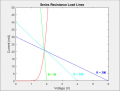#### Janis59

Joined Aug 21, 2017
1,348

The method explained I am using several years telling for schoolboys and schoolgirls the basic concepts of semiconductor biasing, when physics program touches the basics of electronics, but as the engineer I had never used to use this technique - simply not exist any diodes having that Uf=f(if) curve at datasheet except a very raritary minority from very the time apart. So I am telling them, this is concept only; with very difficult implementation but giving a sound view on the principle.

#### atferrari

Joined Jan 6, 2004
4,425
Then, what real common diodes show?

Could you show any graphic from your own record?

And, do datasheets lie?

#### MrChips

Joined Oct 2, 2009
24,178
Finding the Q-point in a circuit requires solving two simultaneous equations.
One equation is the equation of a device that is often non-linear.
The second equation is usually that of a resistive load which is linear.

The second equation is easily derived from circuit analysis.

The first equation is more difficult to come by since it depends on semiconductior physics and information provided in manufacturer's datasheet which could be nonexistent.

So what to do?

It is perfectly ok to use a model that approximates the I-V characteristics of the nonlinear device.

Load line analysis is a simple graphical technique for solving the two simultaneous equations in a very intuitive manner.
If it works and is an effective teaching tool why not use it. I use it all the time for teaching.BTW, the red I-V curve above was determined manually by measuring I over different supply V (with a series resistor) using a red LED.

#### Janis59

Joined Aug 21, 2017
1,348
RE: ""Then, what real common diodes show?""
Of course the table it show
Example? Okay, please find me a u(f)=F from (i(f) graph for any diode in catalog https://www.radiolibrary.ru/reference/diod.html. Dont like, okay, take the catalog http://www.radio-komplekt.ru/component_ref.php?param=diodes
Dont like anyway? Okay, I agree that good firms makes any possible graphs (and then one pays for the "service", but such are not a majority. Most of it worldwide comes cheap, and then with some narrowly selected figures at certain current points instead of graph.

Happily, most of the applications it isnt very much important the precise value of voltage on diode. Enough is with approximate.

#### Wolframore

Joined Jan 21, 2019
2,342
I=Is (e^(qv/kT)-1)

kT/q is about 26 mV at room temp
so the issue is Vd

#### Zeeus

Joined Apr 17, 2019
615
See

To calculate Vo(t), load line is used

#### Janis59

Joined Aug 21, 2017
1,348
RE: Wolframore - yes, this is the common way. It have a correct name the Schokley equation, or in case of bjt the Ebers-Moll equations. However there is one small ugly term labeled "an ideality factor" which never no-one is not showing up how large it is.

•Wolframore

#### MrAl

Joined Jun 17, 2014
8,475
RE: Wolframore - yes, this is the common way. It have a correct name the Schokley equation, or in case of bjt the Ebers-Moll equations. However there is one small ugly term labeled "an ideality factor" which never no-one is not showing up how large it is.
Hi,

That factor is extracted from the physical transistor but can be found in a spice model for the transistor. It is often given as N or NF.
For example, a simple transistor using the least number of parameters for a transistor would have these three:
Is=1e-14
NF=1
BF=100
where Is is the saturation current, NF the forward ideality factor, BF the forward beta.
These are given in a spice model for a given transistor and may vary depending on the transistor.

#### Wolframore

Joined Jan 21, 2019
2,342
Doing some reading on η it seems that it’s a correction factor and one that can not be expressed as it has to do with the shape/flatness of the junction and bandgap. Suffice to say for silicon diodes it is about 1.1 or less and for BJT used as diodes about 1.01. η is always found experimentally. Some components can have more than 2 which would shift our curve quite a bit. For example for GaaN based LEDs η can be quite high. So it appears it’s a correction factor put into the Shockley equation to account for the differences and generally near 1 for silicon and so largely ignored. The factor is significant for germanium but it’s been a long time since I’ve seen those.

I believe our lab tonight we will be building exponential circuits using a BJT and if the results are not as calulated it will be from η. We should be able to identify the value as a corrections to kT/q which we approximate anyhow.

#### Wolframore

Joined Jan 21, 2019
2,342
@Janis59 it seems that N can cause quite a variance especially in BJT where there are 2 junctions!